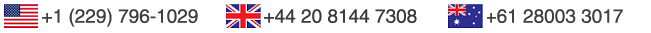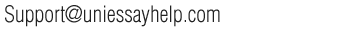Order now# Examine the graph that your spreadsheet produced based upon the calculations.

you were introduced to the concept of exponential functions that can be used to model growth and decay. Recall what you learned in The Number electure. In this exercise, you will use a Microsoft Excel spreadsheet to calculate the exponential growth of a population of your choosing. (If you use the linked spreadsheet below, it is recommended that you consider relatively small populations and not those of entire nations.)

see attachment

In the spreadsheet, perform the following operations:

1. Input a population value into the box next to Initial Population on the spreadsheet. This population should be anything such as the people, animals, microorganisms, or plants.
2. Input a rate of growth for your population into the box next to Rate 1. Since we are interested in a positive annual percent growth rate per year, this number should be greater than zero percent. Be sure to input this number in the form of a decimal.
3. Repeat the above procedure for Rate 2 and Rate 3. Make sure that the values you select differ by two percent. For example, the values 0.01, 0.03, and 0.05 would be good choices.
4. Under the Time (years) column, input three different year values. Make sure that your values increase by a minimum of ten years. For example, 10, 20, 30 years would be good choices. If you want to see a more dramatic change, select a longer time frame such as 10, 50, and 100 years.
5. When you input all of your data, you’ll see that the spreadsheet has performed the calculations for the future size of each population, rate of growth, and time interval. Additionally, this information will be presented graphically in the chart. To draw an exponential curve for each series, do the following:
1. Right click on the middle point for one of the series.
2. Click on add trendline.
3. Under Trendline Options, select Exponential.
4. Click Close
5. Repeat these steps for the other series.

Now that you’ve completed your analysis, it is now time to report the results and examine your findings.

In a Microsoft Word document, respond to the following:

1. Calculate what the future size of the population will be, given a specific initial population, rate of growth, and time interval.

Use the exponential equation: Future value = Present value * exp(rt)

• exp is the base “e”
• r = annual rate of growth expressed as a percent
• t = years

Note: The spreadsheet performed these calculations for you, so you can check the answer you obtain with those in the spreadsheet. In order to perform this calculation, you’ll need to have access to a scientific calculator that will have an ex button in order to perform the exp(rt) calculation. There are a number of free scientific calculators available over the Internet. Additionally, all smartphones have calculator apps that have a scientific mode. Check with your instructor for a list of currently available free options if you do not own a scientific calculator.

1. Repeat the calculation for at least two other values of t; make sure they are at least two years apart from one another. Use the same values you input into the spreadsheet and compare the answers you obtain to those that appear in the spreadsheet.
1. Repeat the calculations using two more selections of population growth rate; ensure that each population growth rate is at least two percent different than the others. Use the same values you input into the spreadsheet and compare the answers you obtain to those that appear in the spreadsheet.
1. Examine the graph that your spreadsheet produced based upon the calculations. Did this graph consist of straight lines or curved lines? Describe the shape of these lines for each growth rate. How did they differ? Why?
2. Explain the implications of growth rate for your population. What do you think will happen over a long period of time if a given population of organisms is allowed to increase without limits? Are there environmental factors that keep populations from growing exponentially unchecked? What would be the impact on environmental resources?
3. Explain the likelihood of your results. Would it be expected that the percent growth rate would stay constant over long periods? Is exponential growth an appropriate assumption for long periods? If not, what other changes in population size might be expected?

Place a similar order with us or any form of academic custom essays related subject and it will be delivered within its deadline. All assignments are written from scratch based on the instructions which you will provide to ensure it is original and not plagiarized. Kindly use the calculator below to get your order cost; Do not hesitate to contact our support staff if you need any clarifications.

Whatever level of paper you need – college, university, research paper, term paper or just a high school paper, you can safely place an order.